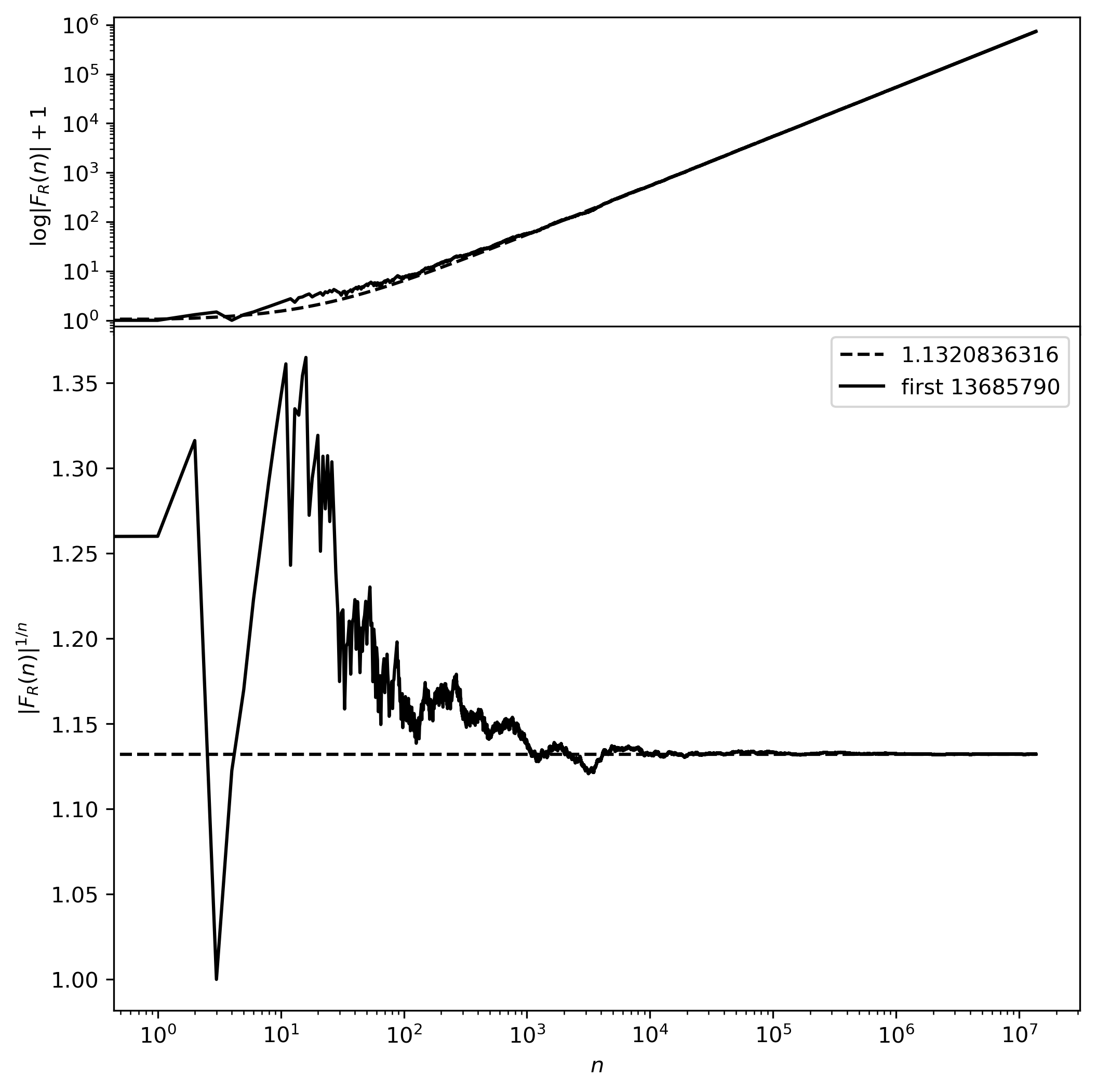# CodingBobby | Random Fibonacci Numbers

Here, I calculated a sequence of random Fibonacci numbers to approximate Viswanath’s constant $$e^{\gamma_f}$$. Below is a Jupyter notbook I used for calculation and plotting. I had time to compute over $$13.5$$ million numbers and found $$e^{\gamma_f}$$ within $$0.0084\,\%$$ error. My final approximation was $$e^{\gamma_f} \approx 1.1320836316$$. If you have $$1.6$$ GB free space on your disk you can download the full list of my computed random Fibonacci numbers with additional information.

Here is the convergence plot in higher resolution:🕸 💍